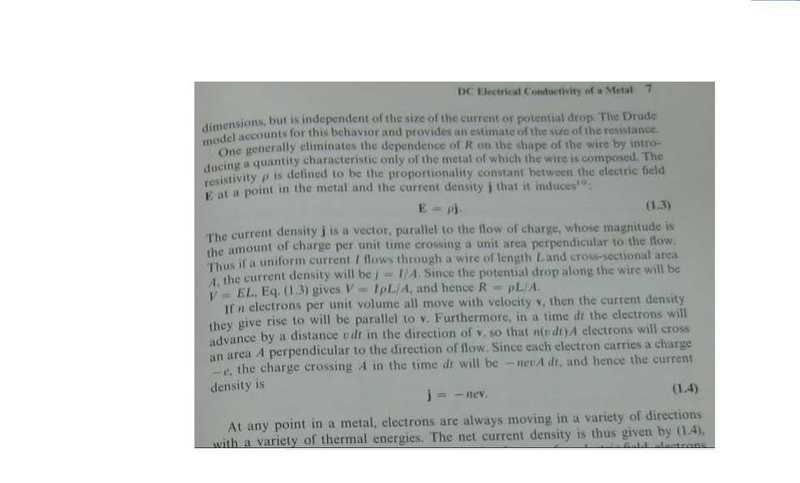# Density definition(semiconductor nightmare 2)

• nhrock3

#### nhrock3drude replaces V=RI with E=rho*j

current density is the amount of charges that crosses a certain area
in an amount of time.
but here they say that if current flows threw a wire of length L etc..
that the current density will be ..

but in the first definition of density there is no length of a wire aspect involved
??

so i can't understand how the length involved here if its not mentioned
in the first definition

Maybe you should keep all your questions to one thread since they all seem to be about solid state physics. To answer your question here: if you multiply a density time a velocity you end up with flux (something/(area)).

list all the units just like we did in the other thread and you'll see. (I'll help you one more time with this.)

$$j = -nev$$

$$j \frac{charge}{seconds}\frac{1}{cm^2}= -n \frac{electrons}{cm^3}e \frac{charge}{electrons}v \frac{cm}{seconds}$$

FYI: Typically, when you work SS problems, you express velocities in (cm/s) not (meters/s). Be careful of unit conversions. Memorize conversions between meters to Angstroms and cm to Angstroms. Trust me on that.

## 1. What is the definition of density in the context of semiconductors?

The density of a semiconductor refers to the number of atoms or molecules per unit volume of the material.

## 2. Why is density an important concept in the study of semiconductors?

Density plays a crucial role in determining the electronic and optical properties of semiconductors, which in turn affect their practical applications in various electronic devices.

## 3. How is density measured in semiconductors?

Density in semiconductors is typically measured using techniques such as X-ray diffraction, transmission electron microscopy, and atomic force microscopy.

## 4. Does density vary between different types of semiconductors?

Yes, density can vary depending on the type of semiconductor material, such as silicon, germanium, or gallium arsenide.

## 5. Can the density of a semiconductor be changed?

Yes, the density of a semiconductor can be altered through processes like doping, which involves introducing impurities to the material, or through external factors like temperature and pressure.